Courses

# Test: 35 Year JEE Previous Year Questions: Solutions

## 30 Questions MCQ Test Class 12 Chemistry 35 Years JEE Mains &Advance Past yr Paper | Test: 35 Year JEE Previous Year Questions: Solutions

Description
This mock test of Test: 35 Year JEE Previous Year Questions: Solutions for JEE helps you for every JEE entrance exam. This contains 30 Multiple Choice Questions for JEE Test: 35 Year JEE Previous Year Questions: Solutions (mcq) to study with solutions a complete question bank. The solved questions answers in this Test: 35 Year JEE Previous Year Questions: Solutions quiz give you a good mix of easy questions and tough questions. JEE students definitely take this Test: 35 Year JEE Previous Year Questions: Solutions exercise for a better result in the exam. You can find other Test: 35 Year JEE Previous Year Questions: Solutions extra questions, long questions & short questions for JEE on EduRev as well by searching above.
QUESTION: 1

### Freezing point of an aqueous solution is (–0.186)°C. Elevationof boiling point of the same solution is Kb = 0.512°C, Kf = 1.86°C, find the increase in boiling point.        

Solution: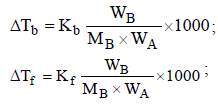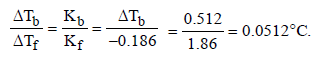QUESTION: 2

### In mixture A and B components show -ve deviation as                

Solution:

In solution containing A and B component showing negative deviation A–A and B–B interactions are weaker than that of A–B interactions. For such solutions.
ΔH = –ve and ΔV = –ve

QUESTION: 3

### If liquids A and B form an ideal solution           

Solution:

When A and B form an ideal solution, ΔHmix = 0

QUESTION: 4

In a 0.2 molal aqueous solution of a weak acid HX the degreeof ionization is 0.3. Taking kf for water as 1.85, the freezingpoint of the solution will be nearest to 

Solution:

ΔTf = Kf × m × i ;
ΔTf = 1.85 × 0.2 × 1.3 = 0.480º C
∴Tf= 0 – 0.480ºC = – 0.480ºC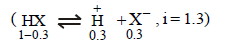QUESTION: 5

A pressure cooker reduces cooking time for food because

Solution:

NOTE : On increasing pressure, the temperature is also increased. Thus in pressure cooker due to increase in pressure the b.p. of water increases

QUESTION: 6

Which one of the following aqueous solutions will exihibithighest boiling point ? 

Solution: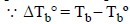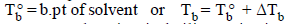NOTE : Elevation in boiling point is a colligative property, which depends upon the no. of particles.Thus greater the number of particles, greater is it elevation and hence greater will be its boiling point.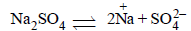Since Na2SO4 has maximum number of particles (3) hence has maximum boiling point.

QUESTION: 7

For which of the following parameters the structural isomersC2H5OH and CH3OCH3 would be expected to have the samevalues?(Assume ideal behaviour) 

Solution:

Gaseous densities of ethanol and dimethyl ether would be same at same temperature and pressure. The heat of vaporisation, V.P. and b.pts will differ due to H-bonding in ethanol.

QUESTION: 8

Which of the following liquid pairs shows a positivedeviation from Raoult’s law ? 

Solution:
QUESTION: 9

Which one of the following statements is FALSE? 

Solution:
QUESTION: 10

Benzene and toluene form nearly ideal solution. At 20°C,the vapour pressure of benzene is 75 torr and that of tolueneis 22 torr. The partial vapour pressure of benzene at 20°C fora solution containing 78 g of benzene and 46 g of toluene intorr is 

Solution:
QUESTION: 11

Equimolar solutions in the same solvent have 

Solution:
QUESTION: 12

Among the following mixtures, dipole-dipole as the majorinteraction, is present in 

Solution:
QUESTION: 13

18 g of glucose (C6H12O6) is added to 178.2 g of water. Thevapour pressure of water for this aqueous solution at 100ºC is 

Solution:
Molecular mass of water = 2*1 + 1*16 = 18 g
For 178.2 g water, nA = 9.9
Molecular mass of glucose = 6*12 + 12*1 + 6*16 = 180 g
For 18 g glucose, nB = 0.1
XB = 0.1/(0.1+9.9) = 0.01
XA = 0.99
For lowering of vapour pressure,
P = p^0A^XA = p^0A(1 – XB)
P = 760(1 – 0.01)
= 760 - 7.6
= 752.4 torr
The correct option is B.
QUESTION: 14

A mixture of ethyl alcohol and propyl alcohol has a vapourpressure of 290 mm at 300 K. The vapour pressure of propylalcohol is 200 mm. If the mole fraction of ethyl alcohol is0.6, its vapour pressure (in mm) at the same temperature willbe 

Solution:
QUESTION: 15

Equal masses of methane and oxygen are mixed in an emptycontainer at 25°C. The fraction of the total pressure exertedby oxygen is 

Solution:
Molar mass of methane = 16.042g mol-1
Molar mass of oxygen = 32.00 g mol-1
Therefore if say, 32g of methane and 32g of oxygen mixed,
there is 2 moles of methane and 1 moles of oxygen.
n= PV/RT
pressure is directly proportional to the number of moles
Oxygen is 1/3 of total number of moles and hence it exerts 1/3 of the total pressure
So my answer would be (D)
QUESTION: 16

A 5.25% solution of a substance is isotonic with a 1.5% solutionof urea (molar mass = 60 g mol–1) in the same solvent. If thedensities of both the solutions are assumed to be equal to 1.0g cm–3, molar mass of the substance will be 

Solution:
QUESTION: 17

At 80° C, the vapour pressure of pure liquid ‘A’ is 520 mmHg and that of pure liquid ‘B’ is 1000 mm Hg. If a mixturesolution of ‘A’ and ‘B’ boils at 80° C and 1 atm pressure, theamount of ‘A’ in the mixture is (1 atm = 760 mm Hg) 

Solution:
QUESTION: 18

The vapour pressure of water at 20° C is 17.5 mm Hg. If 18 gof glucose (C6H12O6) is added to 178.2 g of water at 20° C,the vapour pressure of the resulting solution will be 

Solution:
In solution containing nonvolatile solute,pressure  is directly proportional to its mole fraction.
Psolution = vapour pressure of its pure component * mole fraction in solution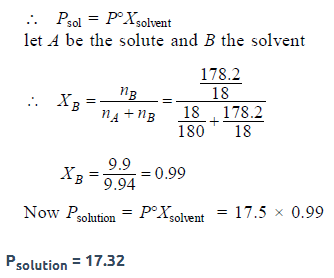QUESTION: 19

A binary liquid solution is prepared by mixing n-heptaneand ethanol. Which one of the following statements iscorrect regarding the behaviour of the solution? 

Solution:

For this solution intermolecular interactions between n-heptane and ethanol aare weaker than n-heptane - nheptane & ethanol-ethanol interactions hence the solution of n-heptane and ethanol is non-ideal and shows positive deviation from Raoult’s law

QUESTION: 20

Two liquids X and Y form an ideal solution. At 300 K, vapourpressure of the solution containing 1 mol of X and 3 mol ofY is 550 mmHg. At the same temperature, if 1 mol of Y isfurther added to this solution, vapour pressure of thesolution increases by 10 mmHg. Vapour pressure ( in mmHg)of X and Y in their pure states will be, respectively: 

Solution:
QUESTION: 21

If sodium sulphate is considered to be completelydissociated into cations and anions in aqueous solution,the change in freezing point of water (ΔTf), when 0.01 molof sodium sulphate is dissolved in 1 kg of water, is(Kf = 1.86 K kg mol–1) 

Solution:
QUESTION: 22

On mixing, heptane and octane form an ideal solution. At 373 K, the vapour pressures of the two liquid components(heptane and octane) are 105 kPa and 45 kPa respectively.Vapour pressure of the solution obtained by mixing 25.0 gof heptane and 35 g of octane will be(molar mass of heptane = 100 g mol–1 and of octane = 114 gmol–1) 

Solution: Using Raoult's law:-Total vapour pressure=(partial vapour pressure of heptane + partial vapour pressure of octane)= P1X1 + P2X2Where X is mole fraction of component
100 g heptane = 1 mole. 25 g heptane = 0.25 moles. 114 g octane = 1 mole35 g octane = 35/114 = 0.307 moles
Mole fraction of heptane = n1/(n1 + n2)=0.25/(0.25+0.307)=0.4488
Mole fraction of octane = n2/(n1 + n2)=0.307/(0.25+0.307)=0.5511
Applying this in Raoult's law105(0.4488)+45(0.5511)You get 72 kPa
QUESTION: 23

A 5.2 molal aqueous solution of methyl alcohol, CH3OH, issupplied. What is the mole fraction of methyl alcohol in thesolution? 

Solution:
QUESTION: 24

Ethylene glycol is used as an antifreeze in a cold climate.Mass of ethylene glycol which should be added to 4 kg ofwater to prevent it from freezing at –6°C will be : (Kf forwater = 1.86 K kg mol–1, and molar mass of ethylene glycol= 62 g mol–1) 

Solution:
QUESTION: 25

The degree of dissociation (a) of a weak electrolyte, AxBy is related to van’t Hoff factor (i) by the expression 

Solution:
QUESTION: 26

The density of a solution prepared by dissolving 120 g ofurea (mol. mass = 60 u) in 1000 g of water is 1.15 g/mL. Themolarity of this solution is : 

Solution:
QUESTION: 27

Kf for water is 1.86 K kg mol–1. If your automobile radiatorholds 1.0 kg of water, how many grams of ethylene glycol(C2H6O2) must you add to get the freezing point of thesolution lowered to –2.8ºC ? 

Solution:
QUESTION: 28

The molarity of a solution obtained by mixing 750 mL of 0.5(M) HCl with 250 mL of 2(M) HCl will be : [JEE M 2013]

Solution:
QUESTION: 29

Consider separate solutions of 0.500 M C2H5OH(aq),0.100 M Mg3(PO4)2 (aq), 0.250 M KBr(aq) and 0.125 MNa3PO4(aq) at 25 C ° . Which statement is true about thesesolutions, assuming all salts to be strong electrolytes?      [JEE M 2014]

Solution:
QUESTION: 30

The vapour pressure of acetone at 20°C is 185 torr. When1.2 g of a non-volatile substance was dissolved in 100 g ofacetone at 20°C, its vapour pressure was 183 torr. The molarmass (g mol–1) of the substance is : [JEE M 2015]

Solution:

Track your progress, build streaks, highlight & save important lessons and more!

### Similar Content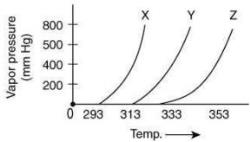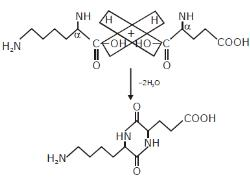### Related tests